## The InDels and Structural Variants detection algorithm - Step 1: Creating Left- and Right breakpoint signatures

In the first step of the InDels and Structural Variants detection algorithm points in the read mapping are identified which have a significant proportion of reads mapped with unaligned ends. There are typically numerous reads with unaligned ends in read mappings -- some are due to structural variants in the sample relative to the reference, others are due to poorly mapped, or poor quality reads. An example is given in figure 25.14. In order to make reliable predictions, attempts must be made to distinguish the unaligned ends caused by noisy read(mappings) from those caused by structural variants, so that the signal from the structural variants comes through as clearly as possible -- both in terms of where the 'significant' unaligned ends are and in terms of what they look like.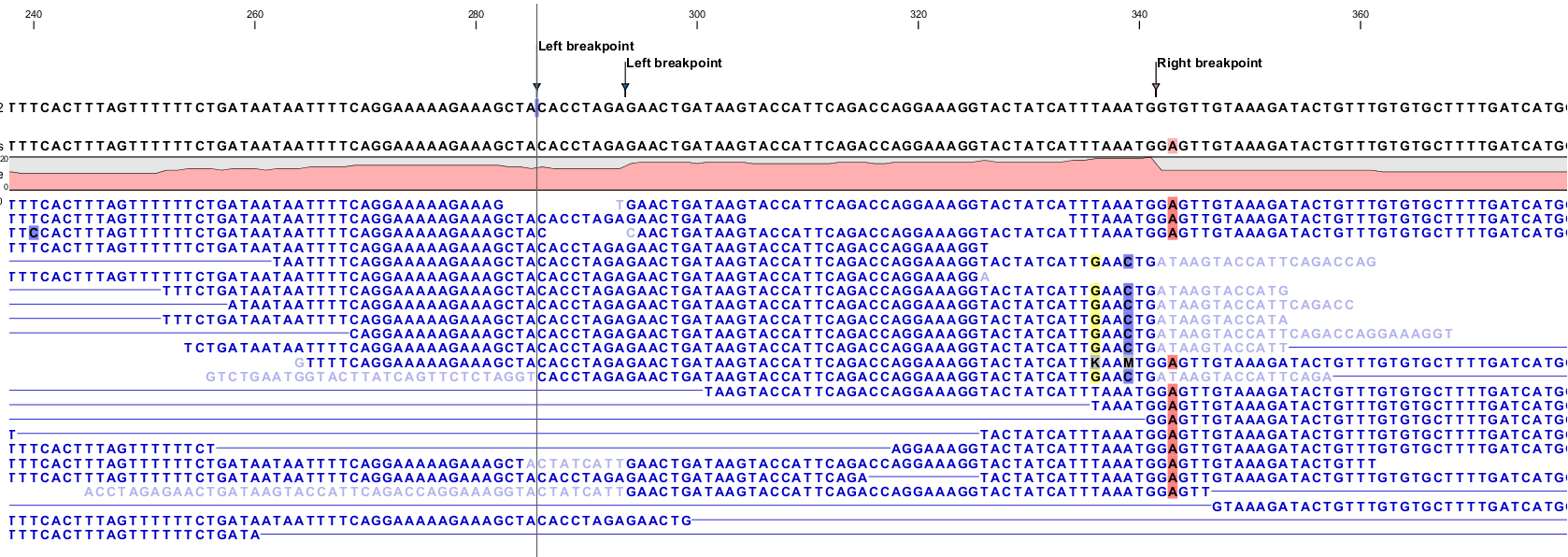Figure 25.14: Example of a read mapping containing unaligned ends with three unaligned end signatures.

To identify positions with a 'significant' portion of 'consistent' unaligned end reads we first estimate 'null-distributions' of the fractions of left and right unaligned end reads at each position in the read mapping, and subsequently use these distributions to identify positions with an 'excess' of unaligned end reads. In these positions we create a Left (LB) or Right (RB) breakpoint signature. To estimate the null-distributions we:

1. Calculate the coverage,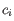, in each position,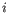of all uniquely mapped reads (Non-specifically mapped reads are ignored. Furthermore, for paired read data sets, only intact paired reads pairs are considered -- broken paired reads are ignored).
2. Calculate the coverage in each position of 'valid' reads with a starting left unaligned end,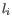(of minimum consensus length 3bp).
3. Calculate the coverage in each position of 'valid' reads with a starting right unaligned end,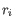(of minimum consensus length 3bp).
We then use the observed fractions of 'Left unaligned ends' (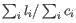) and 'Right unaligned ends' (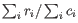) as frequencies in binomial distributions of 'Left unaligned end' and 'Right unaligned end' read fractions. We go through each position in the read mapping and examine it for an excess of left (or right) unaligned end reads: if the probability of obtaining the observed number of left (or right) unaligned ends in a position with the observed coverage, is 'small', a Left breakpoint signature (LB), respectively Right breakpoint signature (RB), is created.

The two user-specified settings 'The P-value threshold' and the 'Maximum number of mismatches' determine which breakpoint signatures the algorithm will detect (see section 25.2.1 and Figure 25.11). The p-value is used as a cutoff in the binomial distributions estimated above: if the probability of obtaining the observed number of left (or right) unaligned ends in a position with the observed coverage, is smaller than the user-specified cut-off, a Left breakpoint signature (LB), respectively Right breakpoint signature (RB), is created. The 'Maximum number of mis-matches' parameter is used to determine which reads are considered 'valid' unaligned end reads. Only reads that have at most this number of mis-matches in their aligned parts are counted. The higher these two values are set, the more breakpoints will be called. The more breakpoints are called, the larger the search space for the Structural variation detection algorithm, and thus the longer the computation time.

In figure 25.14, three unaligned end signatures are shown. The left-most LB signature is called only when the p-value cut-off is chosen high (0.01 as opposed to 0.0001).